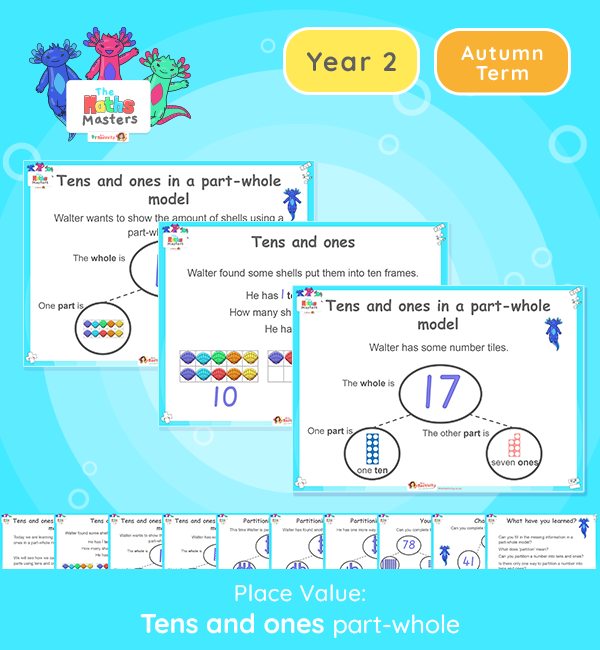# Year 2 | Tens and Ones Using a Part-Whole Model Lesson Presentation## Year 2 White Rose Maths Place Value Resources

This lesson is about splitting wholes into different combinations of tens and ones using a part-whole model

Aligned with the White Rose Maths scheme of work, this Year 2 | Tens and Ones Using a Part-Whole Model Lesson Presentation is fully editable, and is designed for the Year 2 maths curriculum covering the following maths objectives for the autumn term:

Topic/Block: Place Value

White Rose Maths Small Steps: Tens and ones using a part-whole model.

NC Links: Read and write numbers to at least 100 in numerals and words. Recognise the place value of each digit in a two-digit number, Identify, represent and estimate numbers using different representations.

Ready-to-progress criteria: Year 1 Conceptual Prerequisites: Know the 10 ones are equivalent to 1 ten. Know that multiples of 10 are made up from a number of tens.

2-NPV-1 Recognise the place value of each digit in two-digit numbers, and compose and decompose two-digit numbers using standard and non-standard partitioning.

Future Applications: Add and subtract using mental and formal written methods.

TAF Statements: Working towards – Read and write numbers in numerals up to 100. Partition a two-digit number into tens and ones and demonstrate an understanding of place value.

Working At: Partition two digit numbers into different combinations of tens and ones, explaining their thinking verbally, in pictures, or using apparatus.

There are teacher prompts and notes included in the “notes” section of each slide.

Aligned with the order of teaching of the White Rose Maths scheme of work, use this to help your class get to grips with each mathematical concept. This lesson presentation also includes varied fluency activities, problem solving, and mathematical discussion questions too.

Explore our other year 2 place value resources.